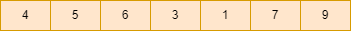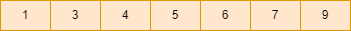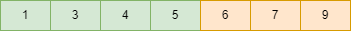# Minimize the sum of minimum and second minimum elements from all possible triplets

## Introduction

This blog will discuss the problem of finding the minimum possible sum of minimum and second minimum of all possible triplets in an array.

### Problem Statement

We are given an array having n elements. Our task is to minimize the sum of minimum and second minimum elements from all possible triplets from the given array. A single element of the given array can only be a part of one triplet.

### Sample examples

Input - 1: a[] = [ 4, 5, 6, 3, 1, 7, 9 ]

Output - 1: 13

Explanation: There are only two possible triplets as there are only 7 elements in the given array.

Triplet1: (1, 3, 9)

Triplet2: (4, 5, 7)

Sum of minimum and second minimum elements of all possible triplets = 1 + 3 + 4 + 5

Input - 2: a[] = [ 4, 5, 6, 7, 8 ,9, 1, 2, 3 ]

Output - 2: 21

Explanation: There are 3 possible triplets as there are 9 elements in the given array

Triplet1: (1,2,9)

Triplet2: (3,4,8)

Triplet3: (5,6,7)

Sum of minimum and second minimum elements of all possible triplets = 1 + 2 + 3 + 4 + 5 + 6 = 21

## Approach

The idea is very simple, count the possible number of triplets from the given array. Now, as we need to minimize the sum of the minimum and second minimum elements for every possible triplet, we calculate the sum of the first k minimum numbers in the given array where k = 2 * number of possible triplets.

To find the sum of the first k minimum elements in the given array, sort the array in non-decreasing order and find the sum of the first k elements.

### Steps of algorithm

• Calculate the possible number of triplets and store it in the possible_triplets variable, where possible_triplets = n/3.
• Declare a variable k and do k = 2 * possible triplets.
• Now, sort the given array, sort(a, a+n).
• Declare a variable ans and initialize it with 0.
• Finally, calculate the sum of the first k elements in the given array and store them in the ans variable.
• Return the value of ans variable.

Let’s understand the above approach with an example:

Given array:• possible_triplets = n/3 = 7/3 = 2
• k = 2*possible_triplets = 2*2 = 4
• Now, sort the given array.• ans = 0
• Calculate the sum of the first k elements.• ans = 1 + 3+ 4 + 5 = 13

Finally, return the value of ans variable.

### Implementation in C++

``````#include<bits/stdc++.h>
using namespace std;

int minm_sum(int a[], int n)
{
int possible_triplets = n / 3;  // possible number of triplets

int k = 2 * possible_triplets;

sort(a, a + n);    // sorting the given array

int ans = 0;

for (int i = 0; i < k; i++)   //first k minimum elements sum
{
ans = ans + a[i];
}
return ans;
}

int main()
{
int a[] = { 4, 5, 6, 8, 7 , 9, 1, 2, 3 }; //given array

int n = sizeof(a) / sizeof(a);    // size of array

cout << minm_sum(a, n);

return 0;
}``````

Output:

``21``

#### Complexity Analysis

Time complexity: We are using the sort function, so the time complexity is O(n*logn), where n is the size of the given array.

Space complexity: O(1) as we have used constant extra space.

Q1. Is STL's sort stable?

Ans: Introsort is typically used by the sort() function. As a result, sort() may or may not preserve the physical order of semantically equivalent values. Mergesort is usually used by the stable sort() function. As a result, stable sort() guarantees that the physical order of semantically equivalent values is preserved.

Q2. What is the C++ STL sort's asymptotic complexity?

Ans: The sorting algorithm is not specified in the language standard and may vary between implementations, but the function's worst-case asymptotic complexity is: when applied to a range of N elements, a call to sort must perform O(N log N) comparisons.

Q3. In C++, how do you find the smallest value in an array?

Ans: One variable, minElement, should be set to the first element of the input array. Traverse the input array from index 0 to N -1 using a loop and compare each element to minElement. Update minElement with the current element if the current element is less than minElement.

## Key Takeaways

This article discussed palindrome and the approach to minimize the sum of minimum and second minimum elements from all possible triplets with examples for a better understanding and its C++ code.

If you are a beginner, interested in coding and want to learn DSA, you can look for our guided path for DSA, which is free!